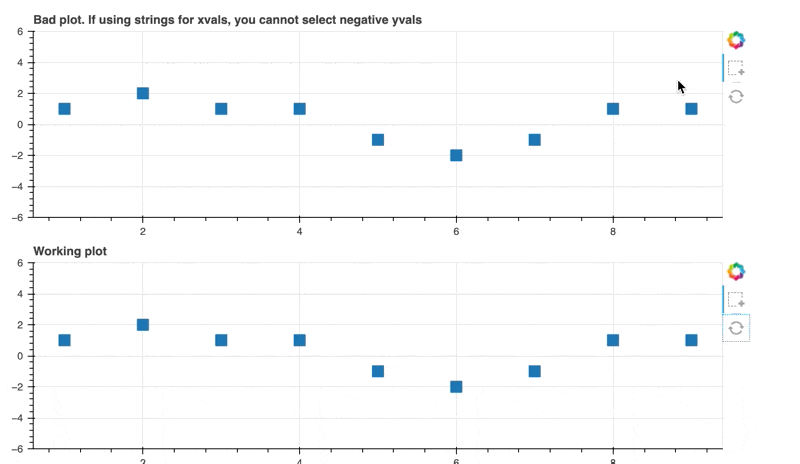# Negative scatter selection

I am getting peculiar behavior when trying to use BoxSelectTool. When I go to select points, it fails to capture the negative points. (1st and 2nd plot)

I determined that this is not specific to the separate renders. If I shift all of the data up, the positive points in both renders are able to be selected. (3rd and 4th plots)

If I need to, I can workaround this problem by adding a large offset and then relabeling the y-tick labels, but I’d rather fix this the proper way if there is some setting that I’m missing.

I’m not sure if this is related, but I also noticed that if I don’t specify the y_range, it will only show positive values. It will scale the y-axis to accommodate whatever positive values I give it, but it does not adjust to contain any negative values. I was able to to view the negative values only after specifying `y_range = [-6,6]`

@alustig3 it is impossible to speculate without a complete
Minimal Reproducible Example and version information (which should always be specified).

Sorry for lack of information. In the process of reducing my code to a minimal reproducible example…I discovered the problem. It’s almost as if creating a minimal reproducible example not only benefits those trying to help, but is also is a good way to debug things in the first place and may prevent the forum from getting spammedIn case future users stumble upon this post with similar difficulties, the problem was that my x-values were a list of numeric strings instead of a list of actual numbers.

Here is a .gif showing the resulting problem of negative numbers not being selected in the first plot. The second plot shows the expected behavior that happens when properly using numbers for the x-values.Below is the code that demonstrates the problem and the solution (shown in the video). I am using Bokeh 2.4.2 with Python 3.10.0

``````import bokeh.plotting as bk
from bokeh.models import BoxSelectTool,ColumnDataSource
from bokeh.layouts import column

trial_strings = ['1','2','3','4','5','6','7','8','9']
trial_nums = [1,2,3,4,5,6,7,8,9]
vals =   [1,2,1,1,-1,-2,-1,1,1]

data={'trials':trial_strings,
'vals': vals }
)
good_source  = ColumnDataSource(
data={'trials':trial_nums,
'vals': vals }
)

width = 800,
height = 250,
tools = "reset",
title = 'Bad plot. If using strings for xvals, you cannot select negative yvals',
y_range = [-6,6],
)
good_plot = bk.figure(
width = 800,
height = 250,
tools = "reset",
title = 'Working plot',
y_range = [-6,6],
)

good_render = good_plot.square('trials','vals',source=good_source, size=12)